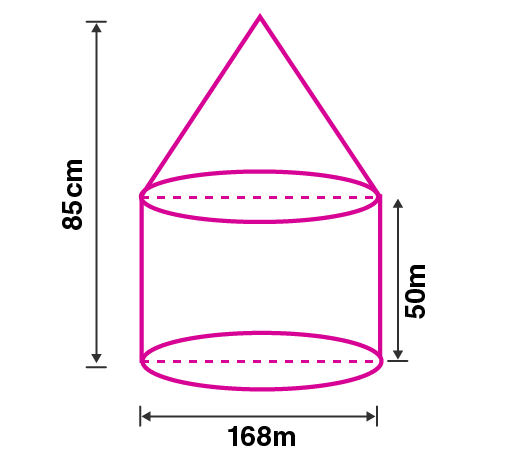Newbie

# An exhibition tent is in the form of a cylinder surmounted by a cone. The height of the tent above the ground is 85 m and the height of the cylindrical part is 50 m. If the diameter of the base is 168 m, find the quantity of canvas required to make the tent. Allow 20% extra for folds and stitching. Give your answer to the nearest m square.

• -1

This question is Based on Mensuration Chapter of M.L Aggarwal book for ICSE BOARD for class 10.
An exhibition tent is in the form of a cylinder surmounted by a cone. The height of the tent above the ground and the height of the cylindrical part is given.
Answer this question I have many doubts.
This is the Question Number 11, Exercise 17.4 of M.L Aggarwal.

Share

1.Given height of the tent above the ground = 85 m

Height of the cylindrical part, H = 50 m

height of the cone, h = 85-50

h = 35 m

Diameter of the base, d = 168 m

Radius of the base of cylindrical part, r = d/2 = 168/2 = 84 m

Radius of cone, r = 84 m

Slant height of the cone, l = √(h2+r2)

= √(352+842)

= √(1225+7056)

= √(8281)

= 91 m

Surface area of tent = curved surface area of cylinder + curved surface area of cone

= 2rH+rl

= r(2H+l)

= (22/7)×84×(2×50+91)

= (22/7)×84×(100+91)

= ((22×84)/7)×191

= (1848/7)×191

= 264×191

= 50424 m2

Adding 20% extra for folds and stitches,

Area of canvas = 50424+ 20% of 50424

= 50424+ (20/100) ×50424

= 50424+ 0.2×50424

= 50424+ 10084.8

= 60508.8 m2

= 60509 m2

Hence the the quantity of canvas required to make the tent is 60509 m2.

• 2